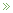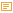• ## What are pseudorandom numbers and what are they used for?

Posted by Swen 25.08.2009

There seems to be a wide split of opinions between theoreticians and practitioners. Namely, some theoreticians define pseudorandomness in terms of complexity theory. As a result, the corresponding constructions are inherently slow and thus rejected by practitioners. This short blog post tries to address some common misconceptions.

Formally, a pseudorandom generator is a deterministic function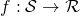which takes a (relatively short) seed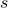and converts it into an element of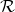. In most cases, we as computer scientists are interested in functions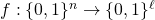. However, there are other reasonable domains, too. For instance, when performing Monte-Carlo integration in the region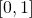, we would be interested in the functions of type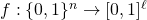.

It is important to note that any function of typeis a pseudorandom generator. However, not all of those functions are equally good for all purposes. There are two main properties that are used to discriminate between various pseudorandom functions. First, we can talk about the efficiency of a pseudorandom generator, i.e., how fast can we compute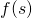. Second, we can talk about the statistical properties of the output.

Before going into the details, let us establish why anyone should use pseudorandom generators at all. There is an interesting controversy in the computer science, as well in statistics. Many algorithms assume that it is possible to use uniformly distributed numbers. For example, Monte-Carlo integration is based on the law of large numbers:whenever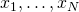are taken independently and uniformly from the range. Similarly, one can provide theoretical bounds for the randomized version of quicksort, provided that we can draw elements uniformly from a set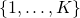. However, computers are mostly made of deterministic parts and it turns out to be really difficult to automatically collect uniformly distributed bits. A design of a device that would solve this problem is far from trivial.

The first official solution to this problem was posed in 1927 when a student of Karl Pearson published a table of random numbers. Later such tables were built by the RAND Corporation. That is, the function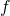was explicitly specified through a big table. Of course, such a table is useful only if it can be used as a "reliable" source of random numbers. In particular, the value of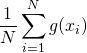should be as close to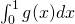as possible. Since there are infinite number of functions, we cannot actually check this condition. Instead, statisticians performed a series of tests on the table to verify that the sequence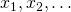looks as close to random as possible.  If we extend this concept properly, we get the modern quantification of pseudorandomness.

Formally, a functionis a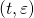-secure pseudorandom generator if for any-time algorithm:where the probabilities are taken over the uniform choices ofand. In more intuitive terms, if you replace the randomnesswithand your algorithm runs in time, then the switch generates no easily observable discrepancies.

As an illustrative example, consider a probabilistic combinatorial search algorithm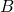that runs in timeand outputs a solution that can be verified in time. In this case, the use of a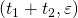-secure pseudorandom generator withininstead of pure randomness would decrease the probability offinding a solution by at most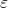. Indeed, otherwise we could construct a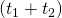-time algorithm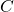that outputs 1, iffinds the solution and 0 otherwise, and use it to discern the pseudorandom generator from true randomness with success at least. This would contradict the fact that we have a true-secure pseudorandom generator.  Similar argument can be proven also for the Monte-Carlo integration algorithm. Note that parametersandcan be arbitrary real numbers. For the combinatorial search algorithm that takes 3 weeks CPU time, you might use a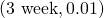-secure pseudorandom generator.

There are essentially two reasonable complaints about the pseudorandom generators. First, since obtaining random bits is hard, we have not solved the problem completely, as we must still get the seed from somewhere. This is indeed a valid problem. For instance, the standard rand() function in C is known to fail the NIST statistical test and thus you might actually observe inconsistencies when using rand() directly or generating a seed for a more complex function with rand(). The latter does not mean that rand() is not a pseudorandom generator, rather that its quality might be low for certain applications. As a complete solution, you would like to get a function. For some tasks, such as Monte-Carlo integration of certain functions the corresponding solution is known (see multidimensional integration and sparse grids).

However, we still do not know how to do it in general, i.e., how to convert randomized algorithms into deterministic ones without significant losses in their performance. The corresponding area of research is known as derandomization. Second, one might argue that we could prove directly that by replacingwith, the performance of the algorithm does not deteriorate much. However, this is not an easy task and it is usually not a valid option for usual mortals.

To summarize, whenever you use a certain functionto extend a random seed in your implementation you actually have to believe that it does not affect performance, i.e.,is a pseudorandom generator with appropriateparameter values. Whether you should use, rand() in C, or something more elaborate, depends on the application.

Posted by Swen @ 8:57 pm

Please note: Comment moderation is enabled and may delay your comment. There is no need to resubmit your comment.

### Calendar

August 2009
M T W T F S S
« Jun   Oct »
12
3456789
10111213141516
17181920212223
24252627282930
31